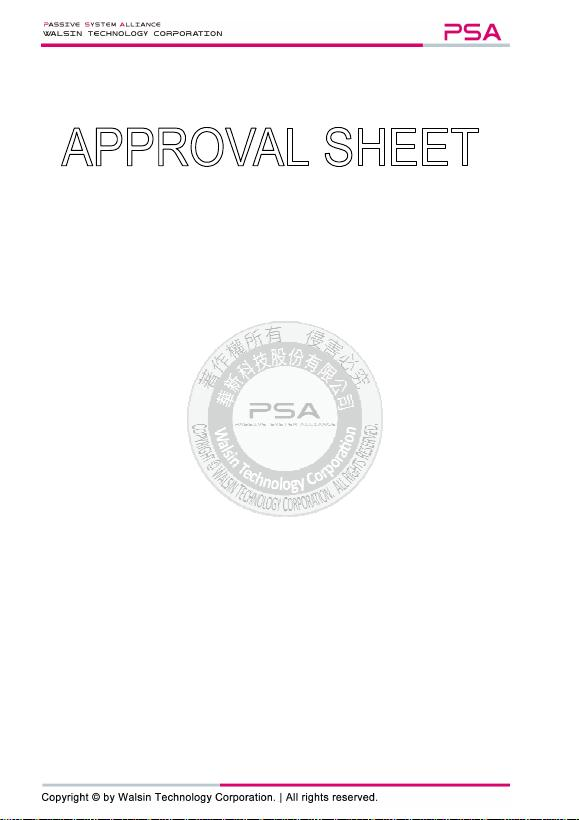Multilayer Ceramic Capacitors Approval Sheet
Page 1 of 22 ASC_General Purpose_001AI_AS Jul. 2020
*Contents in this sheet are subject to change without prior notice.
MULTILAYER CERAMIC CAPACITORS
General Purpose Series (4V to 100V)
0201 to 1812 Sizes
NP0, X7R, Y5V, X6S, X7S & X5R Dielectrics
Halogen Free & RoHS Compliance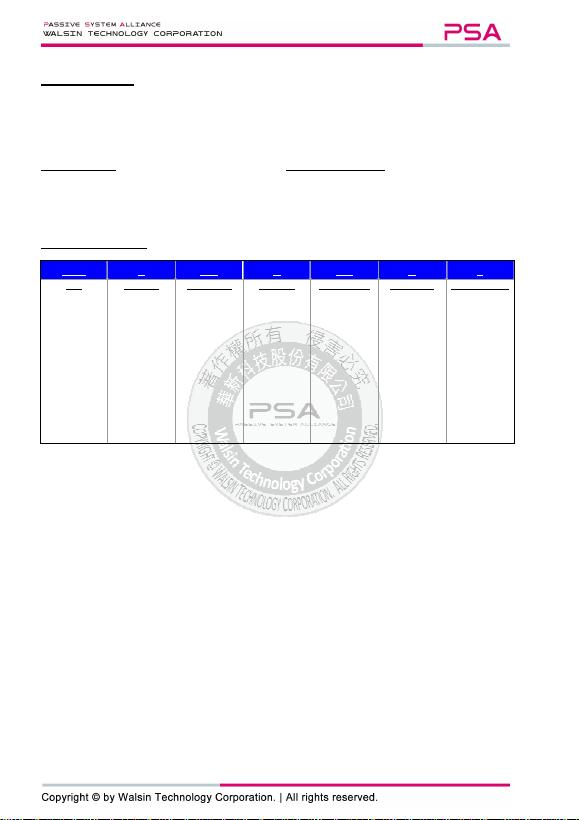Multilayer Ceramic Capacitors Approval Sheet
Page 2 of 22 ASC_General Purpose_001AI_AS Jul. 2020
1. DESCRIPTION
MLCC consists of a conducting material and electrodes. To manufacture a chip-type SMT and achieve miniaturization, high
density and high efficiency, ceramic condensers are used.
WTC’s MLCC is made by NP0, X7R, X6S, X5R and Y5V dielectric material and which provides product with high electrical
precision, stability and reliability.
4. HOW TO ORDER
1206 B 104 K 500 C T
Size
Inch (mm)
0201 (0603)
0402 (1005)
0603 (1608)
0805 (2012)
1206 (3216)
1210 (3225)
1812 (4532)
Dielectric
N=NP0
(C0G)
B=X7R
F=Y5V
X=X5R
S=X6S
A=X7S
Capacitance
Two significant
digits followed by
no. of zeros. And
R is in place of
decimal point.
eg.:
0R5=0.5pF
1R0=1.0pF
104=10x10
4
=100nF
Tolerance
A=±0.05pF
B0.1pF
C0.25pF
D0.5pF
F1%
G2%
J5%
K10%
M20%
Z=-20/+80%
Rated voltage
Two significant
digits followed by
no. of zeros. And
R is in place of
decimal point.
4R0=4 VDC
6R3=6.3 VDC
100=10 VDC
160=16 VDC
250=25 VDC
500=50 VDC
101=100 VDC
Termination
C=Cu/Ni/Sn
Packaging style
T=7” reeled
G=13” reeled
2. FEATURES
a. A wide selection of sizes is available (0201 to 1812).
b. High capacitance in given case size.
c. Capacitor with lead-free termination (pure Tin).
3. APPLICATIONS
a. For general digital circuit.
b. For power supply bypass capacitors.
c. For consumer electronics.
d. For telecommunication.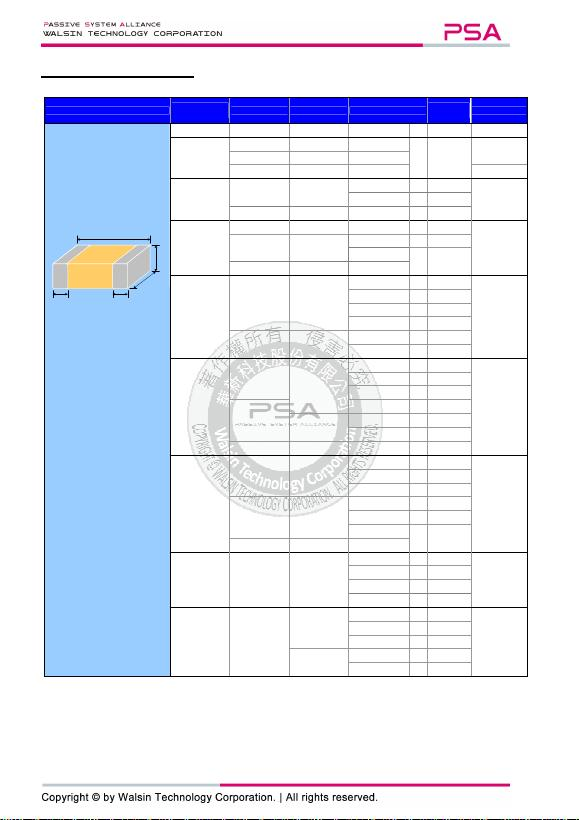Multilayer Ceramic Capacitors Approval Sheet
Page 3 of 22 ASC_General Purpose_001AI_AS Jul. 2020
5. EXTERNAL DIMENSIONS
Outline Size
Inch (mm) L (mm) W (mm) T (mm)/Symbol
Soldering
Method *
M
B
(mm)
1R5 (0402)
0.4±0.02
0.2±0.02
0.2±0.02
V
R
0.10±0.03
0201 (0603)
0.6±0.03
0.3±0.03
0.3±0.03
L
R 0.15±0.05
0.6±0.05
#2
0.3±0.05
#2
0.3±0.05
#2
0.6±0.09
#3
0.3±0.09
#3
0.3±0.09
#3
0.15+0.1/
-
0.05
0402 (1005)
1.00±0.05 0.50±0.05
0.50±0.05
N
R
0.25
+0.05/-0.10
0.50+0.02/
-
0.05
Q
R
1.00±0.20
0.50±0.20
0.5±0.20
E
R
0603 (1608)
1.60±0.10
0.80±0.10
0.80±0.07
S
R / W
0.40±0.15 1.60+0.15/-0.10
0.80+0.15/-0.10
0.50±0.10
H
R / W
0.80+0.15/
-
0.10
X
R / W
1.60±0.20
#1
0.80±0.20
#1
0.8±0.20
#1
0805 (2012)
2.00±0.15 1.25±0.10
0.50±0.10
H
R / W
0.50±0.20
0.60±0.10
A
R / W
0.80±0.10
B
R / W
1.25±0.10
D
R
2.00±0.20 1.25±0.20
0.85±0.10
T
R / W
1.25±0.20
I
R
1206 (3216)
3.20±0.15 1.60±0.15
0.80±0.10
B
R
/ W
0.60±0.20
(0.5±0.25)***
0.95±0.10
C
R
1.25±0.10
D
R
3.20±0.20
1.15±0.15
J
R
1.60±0.20
1.60±0.20
G
R
0.85±0.10
T
R / W
3.20+0.30/-0.10
1.60+0.30/-0.10
1.60+0.30/-0.10
P
R
1210 (3225)
3.20±0.30 2.50±0.20
0.95±0.10
C
R
0.75±0.25
0.85±0.10
T
R
1.25±0.10
D
R
3.20±0.40 2.50±0.30
1.60±0.20
G
R
2.00±0.20
K
R
2.50±0.30
M
R
3.20±0.60
#4
2.50±0.50
#4
2.50±0.50
#4
1808 (4520)
4.50±0.40
(4.5+0.5/-0.3)**
2.03±0.25
1.25±0.10
D
R
0.75±0.25
(0.5±0.25)***
1.40±0.15
F
R
1.60±0.20
G
R
2.00±0.20
K
R
1812 (4532)
4.50±0.40
(4.5+0.5/-0.3)**
3.20±0.30
1.25±0.10
D
R
0.75±0.25
(0.5±0.25)***
1.60±0.20
G
R
2.00±0.20
K
R
3.20±0.40
2.50±0.30
M
R
2.80±0.30
U
R
* R = Reflow soldering process ; W = Wave soldering process.
** For 1808/1812/1825_200V~4000V and safety certificated products.
*** For 1206_1000V, 1808/1812_200V~4000V and safety certificated products.
#1: For 0603/Cap10µF or 0603(
6.3V)/Cap4.7µFor 0603(>10V)/Cap>1µF products.
#2: For 0201/ 0.1uF < Cap < 0.68uF products, Excluding 0201X334~474(
6.3V) & 0201X224(
10V).
#3: For 0201/Cap0.68µF products.
#4: For 1210(100V)/Cap>1µF or 1210(250V)/Cap>0.47µF or 1210(400V~630V)/Cap>0.22µF.
T
W
L
M
B
M
B
Fig. 1 The outline of MLCC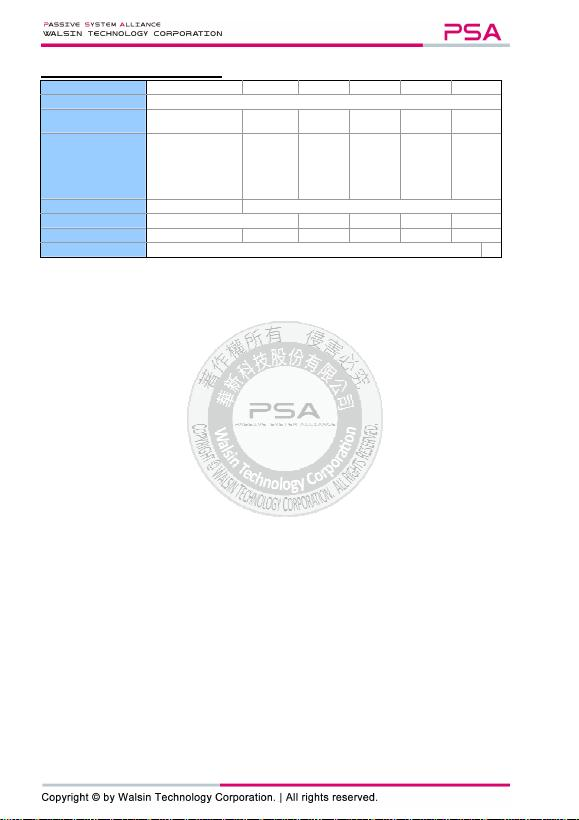Multilayer Ceramic Capacitors Approval Sheet
Page 4 of 22 ASC_General Purpose_001AI_AS Jul. 2020
6. GENERAL ELECTRICAL DATA
Dielectric NP0 X7R Y5V X5R X6S X7S
Size 0201, 0402, 0603, 0805, 1206, 1210, 1812
Capacitance range* 0.1pF to 0.1µF 100pF to 47µF
0.01µF to
100µF 100pF to
220µF 0.1µF to
100µF 1µF to
100µF
Capacitance tolerance**
Cap5pF
#1
:
A (±0.05pF),
B (±0.1pF),
C (±0.25pF)
5pF<Cap<10pF:
C (±0.25pF), D (±0.5pF)
Cap10pF:
F (±1%), G 2%),
J (±5%), K (±10%)
J (±5%),
K (±10%),
M (±20%)
M (±20%),
Z (-20/+80%)
K (±10%),
M (±20%)
K (±10%),
M (±20%)
K (±10%),
M (±20%)
Rated voltage (WVDC) 10V, 16V, 25V, 50V,100V
6.3V, 10V, 16V, 25V, 50V, 100V
Operating temperature -55 to +125°C -25 to +85°C
-55 to +85°C
-55 to +105°C
-55 to +125°C
Capacitance characteristic
±30ppm ±15% +30/-80%
±15% ±22% ±22%
Termination Ni/Sn (lead-free termination)
#1: NP0, 0.1pF product only provide B tolerance; 0603N0R4 provide B&C tolerance; 0603N0R3 only provide C tolerance.
* Measured at the condition of 30~70% related humidity.
NP0: Apply 1.0±0.2Vrms, 1.0MHz±10% for Cap1000pF and 1.0±0.2Vrms, 1.0kHz±10% for Cap>1000pF, 25°C at ambient temperature
X7R/X6S/X5R/X7S: Please refer to page 13 “Reliability test conditions and requirements” for detail.
Y5V: Apply 1.0±0.2Vrms, 1.0kHz±10%, at 20°C ambient temperature.
** Preconditioning for Class II MLCC: Perform a heat treatment at 150±10°C for 1 hour and then leave i n ambient condition for 24±2 hours
before measurement.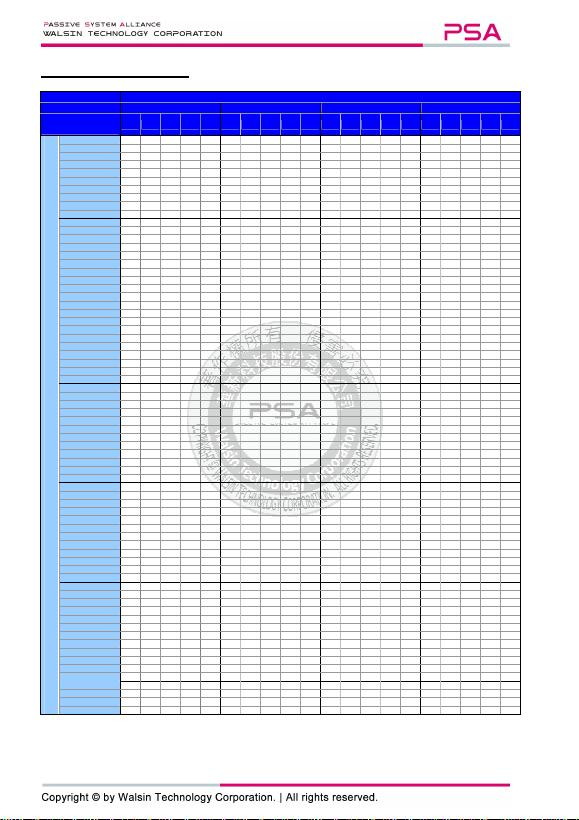Multilayer Ceramic Capacitors Approval Sheet
Page 5 of 22 ASC_General Purpose_001AI_AS Jul. 2020
7. CAPACITANCE RANGE
7-1. NP0 Dielectric 0201, 0402, 0603, 0805 Sizes
DIELECTRIC NP0
SIZE 0201 0402 0603 0805
RATED VOLTAGE
(VDC) 10
16
25
50
100
10
16
25
50
100
10
16
25
50
100
10
16
25
50
100
Capacitance
0.1pF (0R1)
N
N
N
N
0.2pF (0R2)
N
N
N
N
0.3pF (
0R3)
N
N
N
N
S
S
S
S
0.4pF (0R4)
N
N
N
N
S
S
S
S
0.5pF (0R5)
N
N
N
N
N
S
S
S
S
S
A
A
A
A
A
0.6pF (0R6)
N
N
N
N
N
S
S
S
S
S
A
A
A
A
A
0.7pF (0R7)
N
N
N
N
N
S
S
S
S
S
A
A
A
A
A
0.8pF (
0R8)
N
N
N
N
N
S
S
S
S
S
A
A
A
A
A
0.9pF (0R9)
N
N
N
N
N
S
S
S
S
S
A
A
A
A
A
1.0pF (1R0)
N
N
N
N
N
S
S
S
S
S
A
A
A
A
A
1.2pF (1R2)
N
N
N
N
N
S
S
S
S
S
A
A
A
A
A
1.5pF (1R5)
N
N
N
N
N
S
S
S
S
S
A
A
A
A
A
1.8pF (1R8)
N
N
N
N
N
S
S
S
S
S
A
A
A
A
A
2.0pF (2R0)
N
N
N
N
N
S
S
S
S
S
A
A
A
A
A
2.2pF (2R2)
N
N
N
N
N
S
S
S
S
S
A
A
A
A
A
2.7pF (2R7)
N
N
N
N
N
S
S
S
S
S
A
A
A
A
A
3.0pF (3R0)
N
N
N
N
N
S
S
S
S
S
A
A
A
A
A
3.3pF (3R3)
N
N
N
N
N
S
S
S
S
S
A
A
A
A
A
3.9pF (3R9)
N
N
N
N
N
S
S
S
S
S
A
A
A
A
A
4.0pF (4R0)
N
N
N
N
N
S
S
S
S
S
A
A
A
A
A
4.7pF (4R7)
N
N
N
N
N
S
S
S
S
S
A
A
A
A
A
5.0pF (5R0)
N
N
N
N
N
S
S
S
S
S
A
A
A
A
A
5.6pF (5R6)
N
N
N
N
N
S
S
S
S
S
A
A
A
A
A
6.0pF (6R0)
N
N
N
N
N
S
S
S
S
S
A
A
A
A
A
6.8pF (6R8)
N
N
N
N
N
S
S
S
S
S
A
A
A
A
A
7.0pF (7R0)
N
N
N
N
N
S
S
S
S
S
A
A
A
A
A
8.0pF
(8R0)
N
N
N
N
N
S
S
S
S
S
A
A
A
A
A
8.2pF (8R2)
N
N
N
N
N
S
S
S
S
S
A
A
A
A
A
9.0pF (9R0)
N
N
N
N
N
S
S
S
S
S
A
A
A
A
A
10pF (100)
N
N
N
N
N
S
S
S
S
S
A
A
A
A
A
12pF (120)
N
N
N
N
N
S
S
S
S
S
A
A
A
A
A
15pF (150)
N
N
N
N
N
S
S
S
S
S
A
A
A
A
A
18pF (180)
N
N
N
N
N
S
S
S
S
S
A
A
A
A
A
22pF (220)
N
N
N
N
N
S
S
S
S
S
A
A
A
A
A
27pF (270)
N
N
N
N
N
S
S
S
S
S
A
A
A
A
A
33pF (330)
N
N
N
N
N
S
S
S
S
S
A
A
A
A
A
39pF (390)
N
N
N
N
N
S
S
S
S
S
A
A
A
A
A
47pF (470)
N
N
N
N
N
S
S
S
S
S
A
A
A
A
A
56pF (560)
N
N
N
N
N
S
S
S
S
S
A
A
A
A
A
68pF (680)
N
N
N
N
N
S
S
S
S
S
A
A
A
A
A
82pF (820)
N
N
N
N
N
S
S
S
S
S
A
A
A
A
A
100pF (101)
N
N
N
N
N
S
S
S
S
S
A
A
A
A
A
120pF (121)
N
N
N
N
N
S
S
S
S
S
A
A
A
A
A
150pF (151)
N
N
N
N
N
S
S
S
S
S
A
A
A
A
A
180pF (181)
N
N
N
N
N
S
S
S
S
S
A
A
A
A
A
220pF (221)
N
N
N
N
N
S
S
S
S
S
A
A
A
A
A
270pF (271)
N
N
N
N
S
S
S
S
S
A
A
A
A
A
330pF (331)
N
N
N
N
S
S
S
S
S
A
A
A
A
A
390pF (391)
N
N
N
N
S
S
S
S
S
B
B
B
B
B
470pF (471)
N
N
N
N
S
S
S
S
S
B
B
B
B
B
560pF (561)
N
N
N
N
S
S
S
S
S
B
B
B
B
B
680pF (681)
N
N
N
N
S
S
S
S
S
B
B
B
B
B
820pF (821)
N
N
N
N
S
S
S
S
S
B
B
B
B
B
1,000pF (102)
N
N
N
N
S
S
S
S
S
B
B
B
B
B
1,200pF (122)
X
X
X
X
X
*
B
B
B
B
B
1,500pF (152)
X
X
X
X
X
*
B
B
B
B
B
1,800pF (182)
X
X
X
X
B
B
B
B
B
2,200pF (222)
X
X
X
X
B
B
B
B
B
2,700pF (272)
X
X
X
X
D
D
D
D
D
3,300pF (332)
X
X
X
X
D
D
D
D
D
3,900pF (392)
X
X
X
X
D
D
D
D
D
4,700pF (472)
X
X
X
X
D
D
D
D
D
5,600pF (562)
X
*
X
*
X
*
X
*
D
D
D
D
D
6,800pF (682)
X
*
X
*
X
*
X
*
D
D
D
D
D
8,200pF (822)
X
*
X
*
X
*
X
*
D
D
D
D
0.010uF (103)
X
*
X
*
X
*
X
*
D
D
D
D
0.012uF (123)
D
D
D
D
0.01
uF (1
3)
T
*
T
*
T
*
T
*
0.018uF (183)
D
*
D
*
D
*
D
*
0.022uF (223)
D
*
D
*
D
*
D
*
1. The letter in cell is expressed the symbol of product thickness.
2. The letter in cell with “ * ” mark is expressed capacitance tolerance “J” (±5%) only.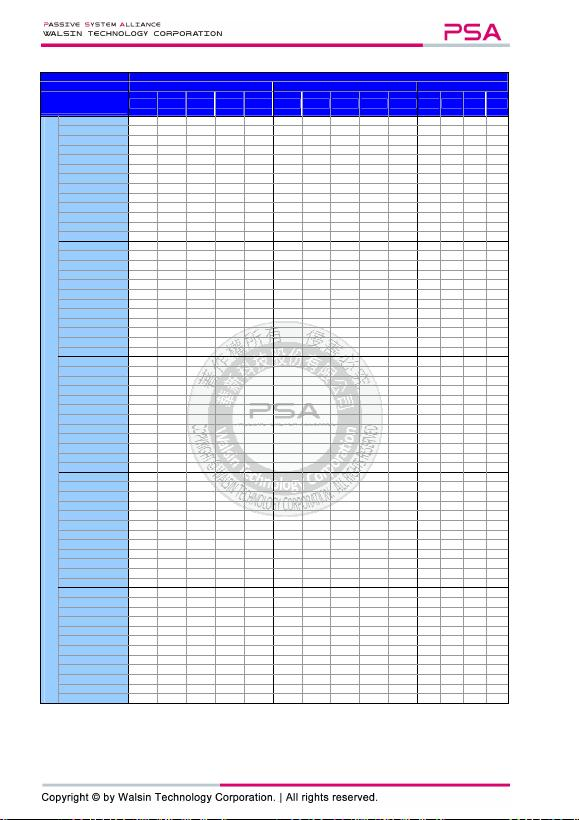Multilayer Ceramic Capacitors Approval Sheet
Page 6 of 22 ASC_General Purpose_001AI_AS Jul. 2020
7-1. NP0 Dielectric 1206, 1210, 1812 Sizes
DIELECTRIC
NP0
SIZE
1206
1210
812
RATED VOLTAGE
(VDC) 10 16 25 50 100
10 16 25 50 100
16
25
50
100
Capacitance
1.0pF (1R0)
1.2pF (1R2)
B
B
B
B
B
1.5pF (1R5)
B
B
B
B
B
1.8pF (1R8)
B
B
B
B
B
2.2pF (2R2)
B
B
B
B
B
2.7pF (2R7)
B
B
B
B
B
3.3pF (3R3)
B
B
B
B
B
3.9pF (3R9)
B
B
B
B
B
4.7pF (4R7)
B
B
B
B
B
5.6pF (5R6)
B
B
B
B
B
6.8pF (6R8)
B
B
B
B
B
8.2pF (8R2)
B
B
B
B
B
10pF (100)
B
B
B
B
B
C
C
C
C
C
D
D
D
D
12pF (120)
B
B
B
B
B
C
C
C
C
C
D
D
D
D
15pF (150)
B
B
B
B
B
C
C
C
C
C
D
D
D
D
18pF (180)
B
B
B
B
B
C
C
C
C
C
D
D
D
D
22pF (220)
B
B
B
B
B
C
C
C
C
C
D
D
D
D
27pF (270)
B
B
B
B
B
C
C
C
C
C
D
D
D
D
33pF (330)
B
B
B
B
B
C
C
C
C
C
D
D
D
D
39pF (390)
B
B
B
B
B
C
C
C
C
C
D
D
D
D
47pF (470)
B
B
B
B
B
C
C
C
C
C
D
D
D
D
56pF (560)
B
B
B
B
B
C
C
C
C
C
D
D
D
D
68pF (680)
B
B
B
B
B
C
C
C
C
C
D
D
D
D
82pF (820)
B
B
B
B
B
C
C
C
C
C
D
D
D
D
100pF (101)
B
B
B
B
B
C
C
C
C
C
D
D
D
D
120pF (121)
B
B
B
B
B
C
C
C
C
C
D
D
D
D
150pF (151)
B
B
B
B
B
C
C
C
C
C
D
D
D
D
180pF (181)
B
B
B
B
B
C
C
C
C
C
D
D
D
D
220pF (221)
B
B
B
B
B
C
C
C
C
C
D
D
D
D
270pF (271)
B
B
B
B
B
C
C
C
C
C
D
D
D
D
330pF (331)
B
B
B
B
B
C
C
C
C
C
D
D
D
D
390pF (391)
B
B
B
B
B
C
C
C
C
C
D
D
D
D
470pF (471)
B
B
B
B
B
C
C
C
C
C
D
D
D
D
560pF (561)
B
B
B
B
B
C
C
C
C
C
D
D
D
D
680pF (681)
B
B
B
B
B
C
C
C
C
C
D
D
D
D
820pF (821)
B
B
B
B
B
C
C
C
C
C
D
D
D
D
1,000pF (102)
B
B
B
B
B
C
C
C
C
C
D
D
D
D
1,200pF (122)
B
B
B
B
B
C
C
C
C
C
D
D
D
D
1,500pF (152)
B
B
B
B
B
C
C
C
C
C
D
D
D
D
1,800pF (182)
B
B
B
B
B
C
C
C
C
C
D
D
D
D
2,200pF (222)
B
B
B
B
B
C
C
C
C
C
D
D
D
D
2,700pF (272)
B
B
B
B
B
C
C
C
C
C
D
D
D
D
3,300pF (332)
B
B
B
B
B
C
C
C
C
C
D
D
D
D
3,900pF (392)
B
B
B
B
B
C
C
C
C
C
D
D
D
D
4,700pF (472)
B
B
B
B
B
C
C
C
C
C
D
D
D
D
5,600pF (562)
B
B
B
B
B
C
C
C
C
C
D
D
D
D
6,800pF (682)
C
C
C
C
C
C
C
C
C
C
D
D
D
D
8,200pF (822)
D
D
D
D
D
C
C
C
C
C
D
D
D
D
0.010µF (103)
D
D
D
D
D
C
C
C
C
C
D
D
D
D
0.012µF (123)
P
P
P
P
P
D
D
D
D
D
D
D
D
D
0.015µF (153)
P
P
P
P
P
D
D
D
D
D
D
D
D
D
0.018µF (183)
P
P
P
P
P
K
K
K
K
K
D
D
D
D
0.022µF (223)
P
P
P
P
P
K
K
K
K
K
D
D
D
D
0.027µF (273)
P
P
P
P
K
K
K
K
K
D
D
D
D
0.033µF (333)
P
P
P
P
T
K
K
K
K
K
D
D
D
D
0.039µF (393)
P
P
P
P
K
K
K
K
K
M
M
M
M
0.0
47
µF (
47
3)
J*
J*
J*
J*
K
K
K
K
K
M
M
M
M
0.0
56
µF (
56
3)
J*
J*
J*
J*
M
M
M
M
0.0
68
µF (
68
3)
G*
G*
G*
G*
M
M
M
M
0.0
82
µF (
82
3)
G*
G*
G*
G*
M
M
M
M
0.
µF (
104
)
G*
G*
G*
G*
M
M
M
M
1. The letter in cell is expressed the symbol of product thickness.
2. The letter in cell with “ * ” mark is expressed capacitance tolerance “J” (±5%) only.